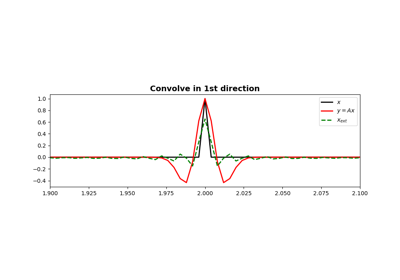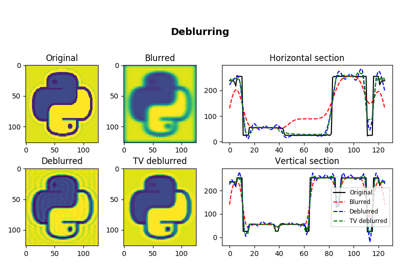# pylops.signalprocessing.Convolve2D¶

class pylops.signalprocessing.Convolve2D(N, h, dims, offset=(0, 0), nodir=None, dtype='float64')[source]

2D convolution operator.

Apply two-dimensional convolution with a compact filter to model (and data) along a pair of specific directions of a two or three-dimensional array depending on the choice of nodir.

Parameters: N : int Number of samples in model h : numpy.ndarray 2d compact filter to be convolved to input signal dims : list Number of samples for each dimension offset : tuple, optional Indeces of the center of the compact filter nodir : int, optional Direction along which convolution is NOT applied (set to None for 2d arrays) dtype : str, optional Type of elements in input array.

Notes

The Convolve2D operator applies two-dimensional convolution between the input signal $$d(t,x)$$ and a compact filter kernel $$h(t,x)$$ in forward model:

$y(t,x) = \int_{-\inf}^{\inf}\int_{-\inf}^{\inf} h(t-\tau,x-\chi) d(\tau,\chi) d\tau d\chi$

This operation can be discretized as follows

$y[i,n] = \sum_{j=-\inf}^{\inf} \sum_{m=-\inf}^{\inf} h[i-j,n-m] d[j,m]$

as well as performed in the frequency domain.

$Y(f, k_x) = \mathscr{F} (h(t,x)) * \mathscr{F} (d(t,x))$

Convolve2D operator uses scipy.signal.convolve2d that automatically chooses the best domain for the operation to be carried out.

As the adjoint of convolution is correlation, Convolve2D operator applies correlation in the adjoint mode.

In time domain:

$y(t,x) = \int_{-\inf}^{\inf}\int_{-\inf}^{\inf} h(t+\tau,x+\chi) d(\tau,\chi) d\tau d\chi$

or in frequency domain:

$y(t, x) = \mathscr{F}^{-1} (H(f, k_x)^* * X(f, k_x))$
Attributes: shape : tuple Operator shape explicit : bool Operator contains a matrix that can be solved explicitly (True) or not (False)

Methods

 __init__(self, N, h, dims[, offset, nodir, …]) Initialize this LinearOperator. adjoint(self) Hermitian adjoint. cond(self, \*\*kwargs_eig) Condition number of linear operator. conj(self) Complex conjugate operator div(self, y[, niter]) Solve the linear problem $$\mathbf{y}=\mathbf{A}\mathbf{x}$$. dot(self, x) Matrix-matrix or matrix-vector multiplication. eigs(self[, neigs, symmetric, niter]) Most significant eigenvalues of linear operator. matmat(self, X) Matrix-matrix multiplication. matvec(self, x) Matrix-vector multiplication. rmatvec(self, x) Adjoint matrix-vector multiplication. transpose(self) Transpose this linear operator.

## Examples using pylops.signalprocessing.Convolve2D¶Convolution04. Image deblurring